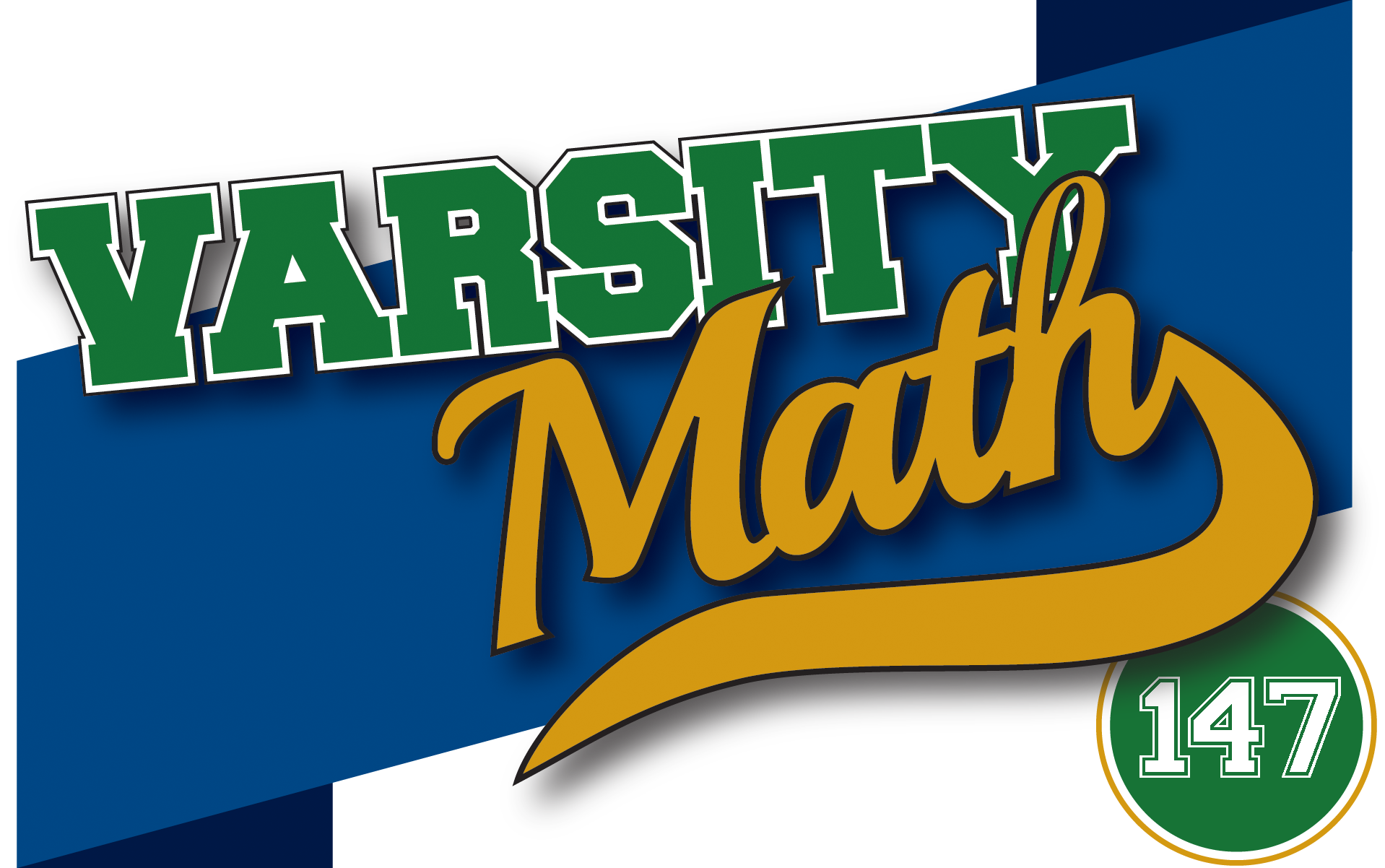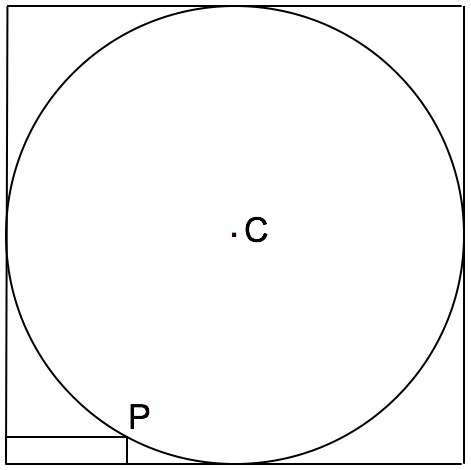## ________________

Welcome to Varsity Math, the weekly math puzzle column by the National Museum of Mathematics and featured each weekend in the Wall Street Journal.

## ________________

### Alternative Sum and Product

The sum of the digits from 1 to 9 is 45 and their product is 9 factorial = 1 × 2 × 3 × 4 × 5 × 6 × 7 × 8 × 9 = 362,880.

Find another set of nine single-digit numbers with a sum of 45 and a product of 9 factorial. Digits may be repeated.### Math Quiz

Aileen, Brian, Laurie and Sue have just received the results of a math quiz they took. Each problem correctly solved earns the same number of points.

Aileen: Our average was half a perfect score.

Brian: I got three wrong.

Laurie: I got nine correct.

Sue: I got eleven wrong.

How many problems did Aileen get correct?

## Solutions to week 146

In Mailing Problem, the coach’s package had a volume of 360 with dimensions (9, 8, 5). Prior to that, the coach had considered a box with dimensions of (10, 9, 4). In Pool Party, the coach’s yard is 34 feet by 34 feet.